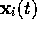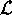Next: The Lagrangian Up: The Dynamics of Galaxies Previous: Hamilton's principle

# Equations of Motion

Let us now return to the specific system dealt with in this thesis; i.e. a set of particles i (galaxies) of mass, expressed in comoving coordinates(see equation (2.5)) in an expanding universe with a mean mass density. The goal of this section is to determine the equations of motion for these particles using Hamilton's principle from the previous section, but first, we need an expression for the scalar function, the Lagrangian.

Trond Hjorteland
Mon Jul 5 02:59:28 MET DST 1999